# Maths InequalitiesPage 1

#### WATCH ALL SLIDES

Slide 1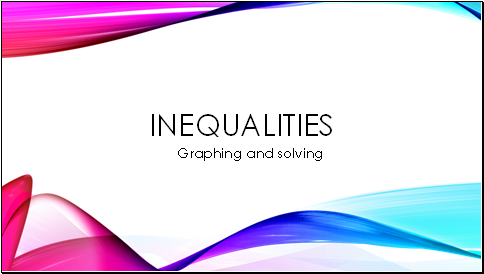Inequalities

Graphing and solving

Slide 2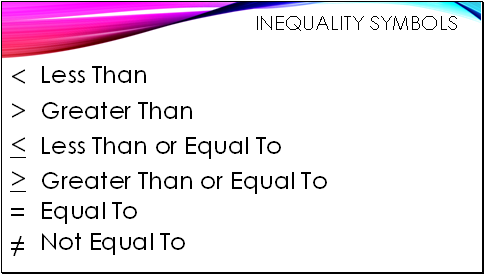## Inequality Symbols

<

>

<

>

=

Less Than

Greater Than

Less Than or Equal To

Greater Than or Equal To

Equal To

Not Equal To

Slide 3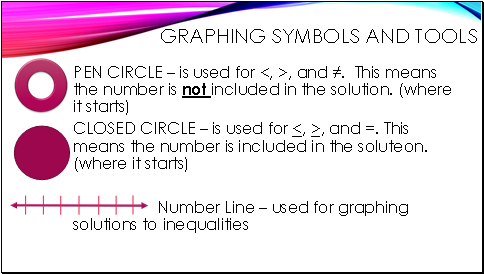## Graphing Symbols and Tools

PEN CIRCLE – is used for <, >, and ≠. This means the number is not included in the solution. (where it starts)

CLOSED CIRCLE – is used for <, >, and =. This means the number is included in the soluteon. (where it starts)

Number Line – used for graphing solutions to inequalities

Slide 4## How to Graph

x > 5

Where does it start?

What type of circle will be used?

Which direction will the arrow go?

x < 3

Where does it start?

What type of circle will be used?

Which direction will the arrow go?

x ≠ 4

Where does it start?

What type of circle will be used?

Which direction will the arrow go?

Slide 5## Now you graph these

x < 10 y > 4 z = 5 a ≠ 6 b ≠ - 8 c < -2 d > -3

Slide 6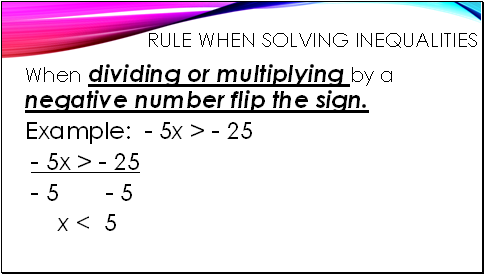## Rule when solving inequalities

When dividing or multiplying by a negative number flip the sign.

Example: - 5x > - 25

- 5x > - 25

- 5 - 5

x < 5

Slide 7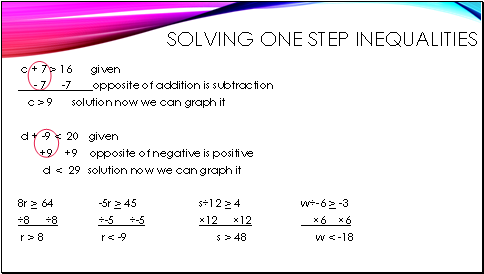## Solving one step inequalities

c + 7 > 16 given

- 7 -7 opposite of addition is subtraction

c > 9 solution now we can graph it

d + -9 < 20 given

+9 +9 opposite of negative is positive

d < 29 solution now we can graph it

8r > 64

÷8 ÷8

r > 8

-5r > 45

÷-5 ÷-5

r < -9

s÷12 > 4

×12 ×12

s > 48

w÷-6 > -3

×6 ×6

w < -18

Slide 8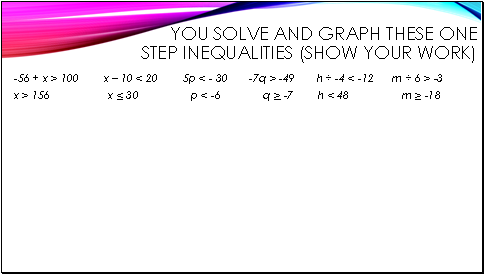## You solve and Graph these one step inequalities (show your work)

-56 + x > 100 x – 10 < 20 5p < - 30 -7q > -49 h ÷ -4 < -12 m ÷ 6 > -3

x > 156 x < 30 p < -6 q > -7 h < 48 m > -18

Slide 9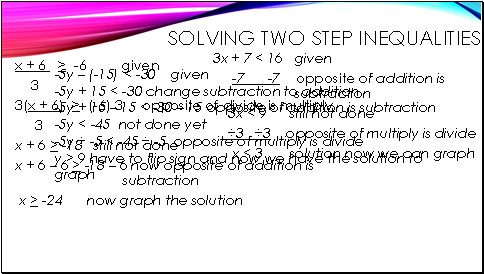## Solving two step inequalities

3x + 7 < 16 given

-7 -7 opposite of addition is subtraction

Go to page:
1  2